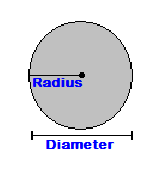CalcuNation

# Circle Circumference Calculator

## Find the circumference of a circle, or perimeter of a circle, by using this Circle Circumference Calculator.Fill in circle radius to find the circumference.

### What is the circumference of a circle?

The circumference is the total distance around a circle.

To find the circumference of a circle, use this circle
circumference formula:
Circle Circumference = 2 x Pi x r

Pi = 3.1416
r = radius of the circle

The radius is a measurement of length.

Example: The circumference of a circle with a radius(r) of 3 inches is:
2 x 3.1416 x 3

Calculated out this gives a circumference of 18.8496 Inches.

Other Calculators of Interest:
Circle Area Calculator, Sphere Area Calculator, Sphere Volume Calculator

### \$100 Promotion

Win \$100 towards teaching supplies! We want to see your websites and blogs.Enter Here

### Calculator Popups

Scientific Calculator
Simple Calculator

### Calculator Ideas

We use your calculator ideas to create new and useful online calculators.
Submit Calculator Idea